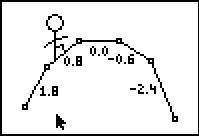••• ##### Device
• TI-83 Plus Family
• TI-84 Plus
• TI-84 Plus Silver Edition
• ##### Software

LearningCheck™ Creator

# Algebra 1: Math Man On The Slopes

by Texas Instruments - Bell Ringer Lesson#### Overview

Students practice identifying slopes with informal pictures, and can self-check their understanding with one of the measurement tools.

#### Key Steps

•Students will visually estimate slope by connecting slope to a man skiing down a hill to reinforce the difference between positive, negative, and zero slope. Then students are given a formation of the big dipper, which has different slopes. They estimate which slope fits which line.

•Students are to change a line so that it has a given slope. They can visually estimate the slope first, and then use the rise/run triangle to specifically place the point. The teacher should follow up with a discussion on y-intercept and the equation of a line in slope-intercept form.

•Students will asses their understanding of slope and y-intercept using self-check multiple choice questions in a provided on the worksheet or in a LearningCheck file.